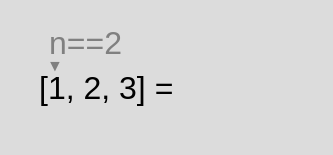Javascript is disabled which is ok, but some features may not work properly. I'll teach you 7 Array methods. (with animations!) I’ll teach you 7 Array methods. (with animations!) | Fredy.dev A blog about programming

# I'll teach you 7 Array methods. (with animations!)

Posted on September 20, 2022.

## map

Creates a new array populated with the results of a provided function on every element.

``````[1, 2, 3].map( n => n * 2 ); // [2, 4, 6]
``````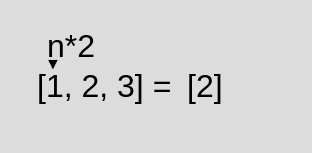## filter

Creates a Copy of a portion of a given array, filtered down to just the elements from the given array that pass the test, keep in mind that changes of your first array will affect your copy.

``````[1,2,3].filter( n => n !== 2 ); // [1,3]
``````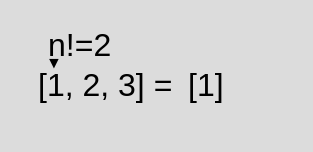## find

Stops and returns the first element in the provided array that satisfies the provided testing function, otherwise returns undefined.

``````[1,2,3].find( n => n == 2 ) // 2
``````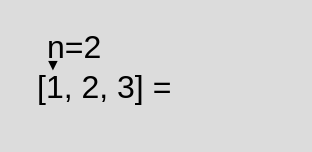## findIndex

Returns the index of the first element that satisfies the provided testing function. Otherwise -1 is returned.

``````[1,2,3].findIndex( n => n == 2) // 1
``````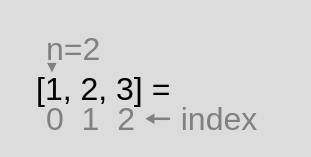## fill

Changes all elements in an array to a static value, from a start index (default 0) to an end index (default array.length). It returns the modified array.

``````[1,2,3].fill('Txt', 1,2) // [1,'Txt', 3]
``````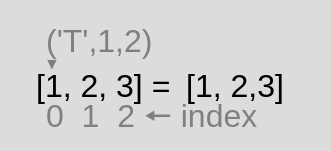## every

Tests all elements in the array. After finished returns a Boolean value

``````[2,2,2].every( n => n == 2 ) // true
``````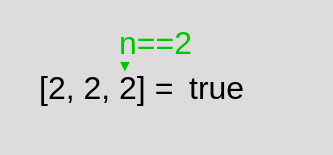## some

Tests whether at least one element in the array passes the test implemented by the provided function.

``````[1,2,3].some( n => n == 2) // true
``````